Wave Optics

# Young’s Double Slit Experiment

OpenStaxCollege

### Learning Objectives

• Explain the phenomena of interference.
• Define constructive interference for a double slit and destructive interference for a double slit.

Although Christiaan Huygens thought that light was a wave, Isaac Newton did not. Newton felt that there were other explanations for color, and for the interference and diffraction effects that were observable at the time. Owing to Newton’s tremendous stature, his view generally prevailed. The fact that Huygens’s principle worked was not considered evidence that was direct enough to prove that light is a wave. The acceptance of the wave character of light came many years later when, in 1801, the English physicist and physician Thomas Young (1773–1829) did his now-classic double slit experiment (see [link]).

Young’s double slit experiment. Here pure-wavelength light sent through a pair of vertical slits is diffracted into a pattern on the screen of numerous vertical lines spread out horizontally. Without diffraction and interference, the light would simply make two lines on the screen.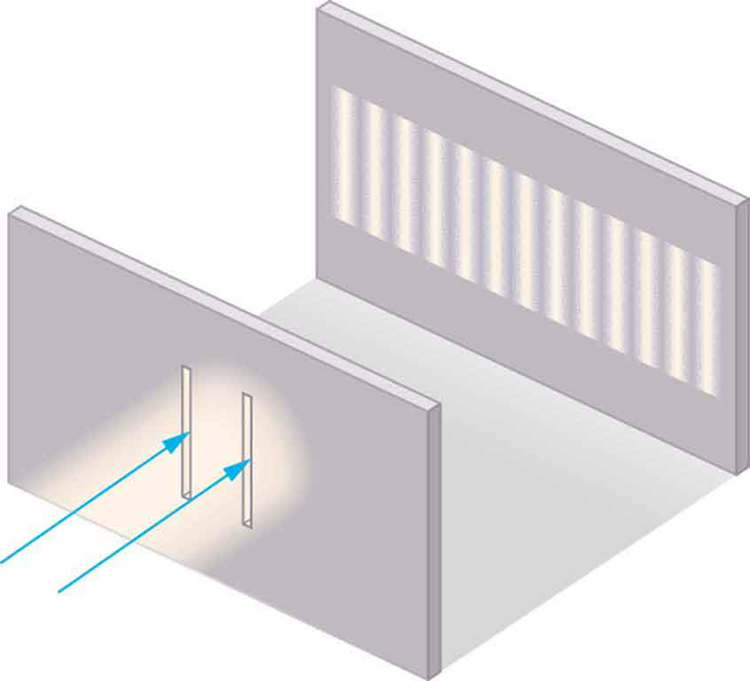Why do we not ordinarily observe wave behavior for light, such as observed in Young’s double slit experiment? First, light must interact with something small, such as the closely spaced slits used by Young, to show pronounced wave effects. Furthermore, Young first passed light from a single source (the Sun) through a single slit to make the light somewhat coherent. By coherent, we mean waves are in phase or have a definite phase relationship. Incoherent means the waves have random phase relationships. Why did Young then pass the light through a double slit? The answer to this question is that two slits provide two coherent light sources that then interfere constructively or destructively. Young used sunlight, where each wavelength forms its own pattern, making the effect more difficult to see. We illustrate the double slit experiment with monochromatic (single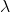) light to clarify the effect. [link] shows the pure constructive and destructive interference of two waves having the same wavelength and amplitude.

The amplitudes of waves add. (a) Pure constructive interference is obtained when identical waves are in phase. (b) Pure destructive interference occurs when identical waves are exactly out of phase, or shifted by half a wavelength.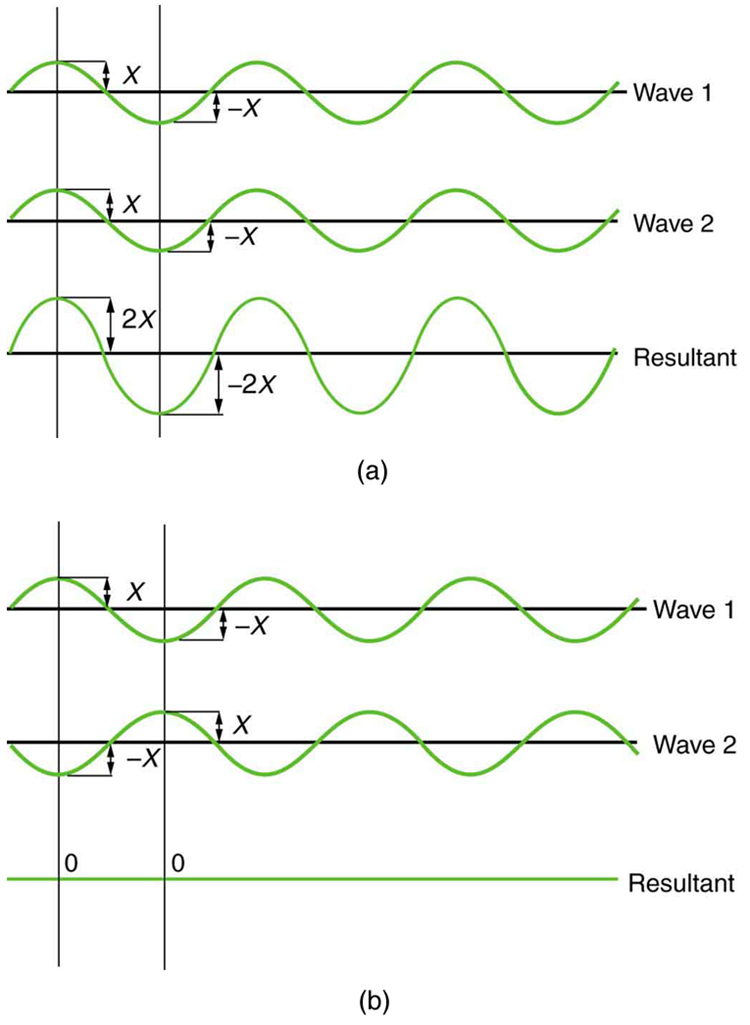When light passes through narrow slits, it is diffracted into semicircular waves, as shown in [link](a). Pure constructive interference occurs where the waves are crest to crest or trough to trough. Pure destructive interference occurs where they are crest to trough. The light must fall on a screen and be scattered into our eyes for us to see the pattern. An analogous pattern for water waves is shown in [link](b). Note that regions of constructive and destructive interference move out from the slits at well-defined angles to the original beam. These angles depend on wavelength and the distance between the slits, as we shall see below.

Double slits produce two coherent sources of waves that interfere. (a) Light spreads out (diffracts) from each slit, because the slits are narrow. These waves overlap and interfere constructively (bright lines) and destructively (dark regions). We can only see this if the light falls onto a screen and is scattered into our eyes. (b) Double slit interference pattern for water waves are nearly identical to that for light. Wave action is greatest in regions of constructive interference and least in regions of destructive interference. (c) When light that has passed through double slits falls on a screen, we see a pattern such as this. (credit: PASCO)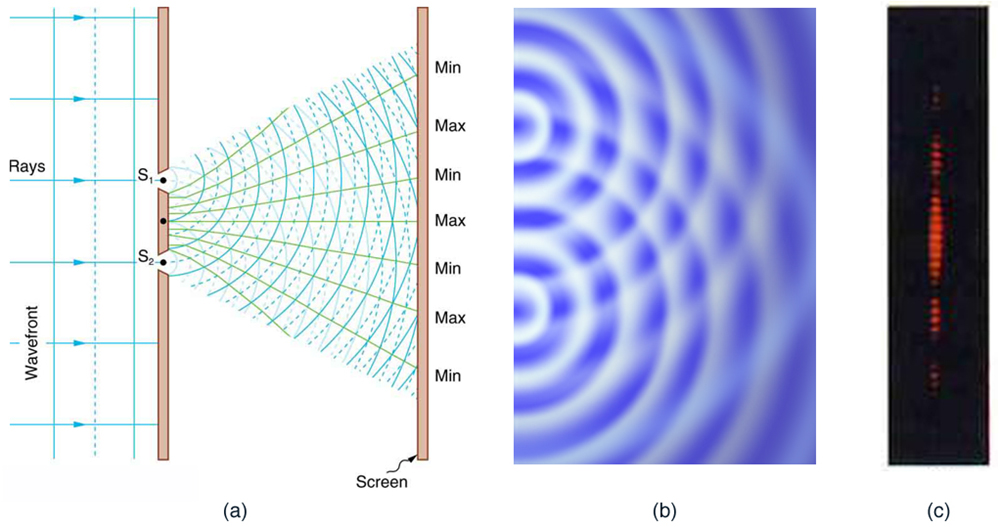To understand the double slit interference pattern, we consider how two waves travel from the slits to the screen, as illustrated in [link]. Each slit is a different distance from a given point on the screen. Thus different numbers of wavelengths fit into each path. Waves start out from the slits in phase (crest to crest), but they may end up out of phase (crest to trough) at the screen if the paths differ in length by half a wavelength, interfering destructively as shown in [link](a). If the paths differ by a whole wavelength, then the waves arrive in phase (crest to crest) at the screen, interfering constructively as shown in [link](b). More generally, if the paths taken by the two waves differ by any half-integral number of wavelengths [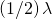,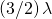,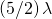, etc.], then destructive interference occurs. Similarly, if the paths taken by the two waves differ by any integral number of wavelengths (,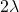,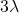, etc.), then constructive interference occurs.

Take-Home Experiment: Using Fingers as Slits

Look at a light, such as a street lamp or incandescent bulb, through the narrow gap between two fingers held close together. What type of pattern do you see? How does it change when you allow the fingers to move a little farther apart? Is it more distinct for a monochromatic source, such as the yellow light from a sodium vapor lamp, than for an incandescent bulb?

Waves follow different paths from the slits to a common point on a screen. (a) Destructive interference occurs here, because one path is a half wavelength longer than the other. The waves start in phase but arrive out of phase. (b) Constructive interference occurs here because one path is a whole wavelength longer than the other. The waves start out and arrive in phase.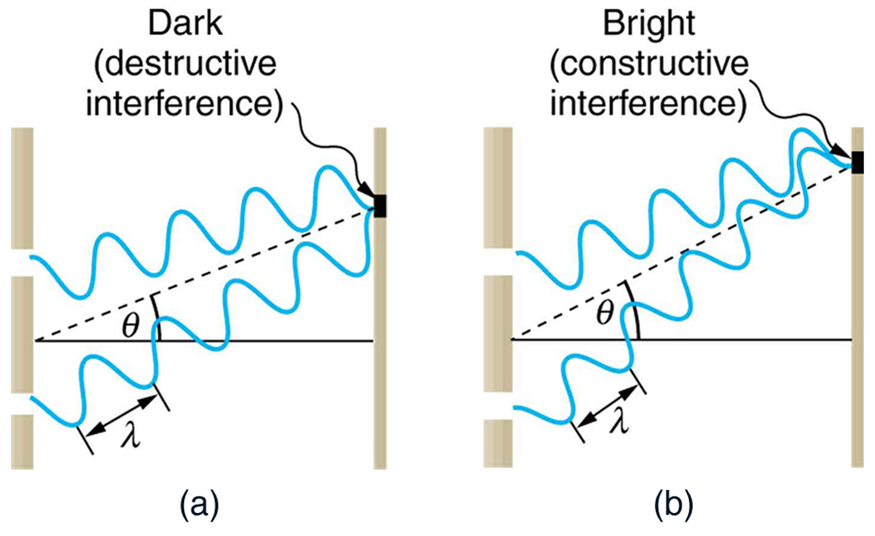[link] shows how to determine the path length difference for waves traveling from two slits to a common point on a screen. If the screen is a large distance away compared with the distance between the slits, then the angle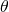between the path and a line from the slits to the screen (see the figure) is nearly the same for each path. The difference between the paths is shown in the figure; simple trigonometry shows it to be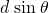, where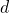is the distance between the slits. To obtain constructive interference for a double slit, the path length difference must be an integral multiple of the wavelength, or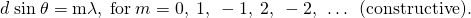Similarly, to obtain destructive interference for a double slit, the path length difference must be a half-integral multiple of the wavelength, or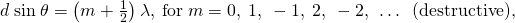whereis the wavelength of the light,is the distance between slits, andis the angle from the original direction of the beam as discussed above. We call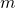the order of the interference. For example,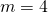is fourth-order interference.

The paths from each slit to a common point on the screen differ by an amount, assuming the distance to the screen is much greater than the distance between slits (not to scale here).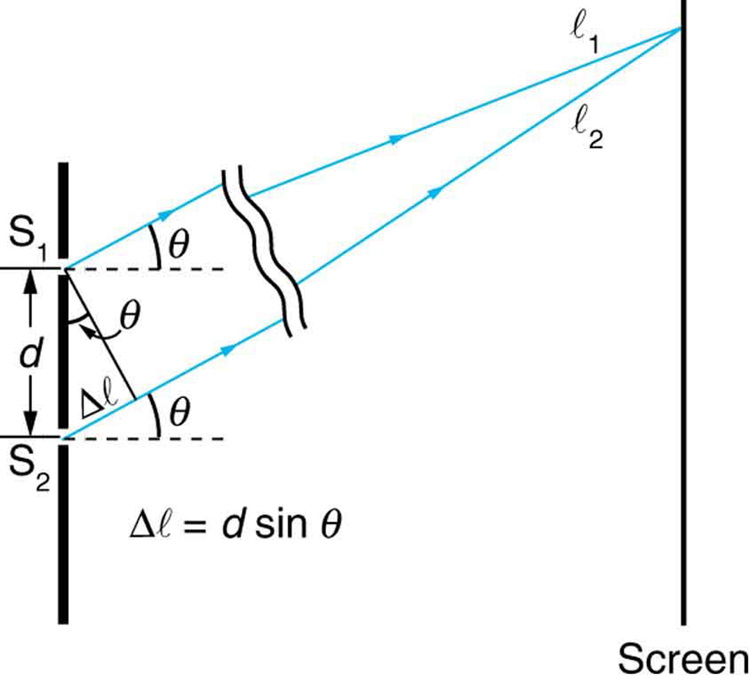The equations for double slit interference imply that a series of bright and dark lines are formed. For vertical slits, the light spreads out horizontally on either side of the incident beam into a pattern called interference fringes, illustrated in [link]. The intensity of the bright fringes falls off on either side, being brightest at the center. The closer the slits are, the more is the spreading of the bright fringes. We can see this by examining the equation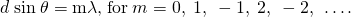For fixedand, the smalleris, the largermust be, since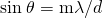.

This is consistent with our contention that wave effects are most noticeable when the object the wave encounters (here, slits a distanceapart) is small. Smallgives large, hence a large effect.

The interference pattern for a double slit has an intensity that falls off with angle. The photograph shows multiple bright and dark lines, or fringes, formed by light passing through a double slit.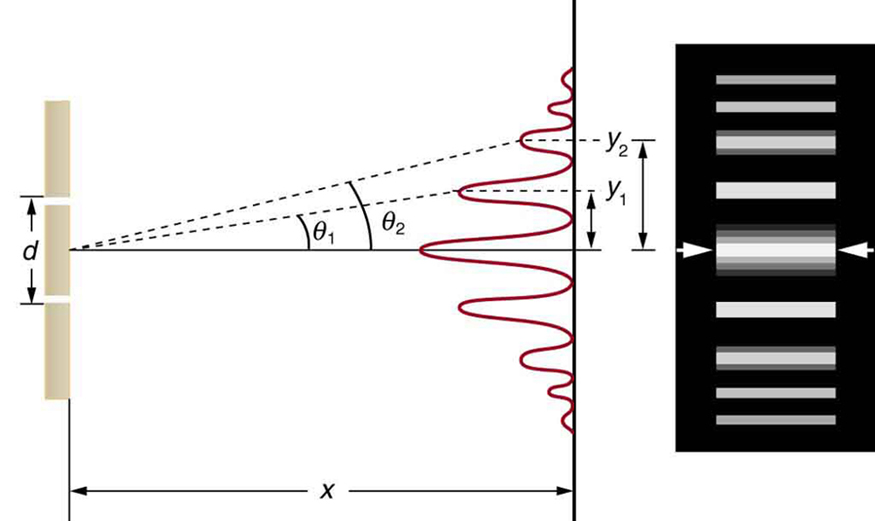Finding a Wavelength from an Interference Pattern

Suppose you pass light from a He-Ne laser through two slits separated by 0.0100 mm and find that the third bright line on a screen is formed at an angle of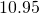relative to the incident beam. What is the wavelength of the light?

Strategy

The third bright line is due to third-order constructive interference, which means that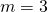. We are given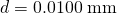and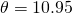. The wavelength can thus be found using the equation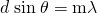for constructive interference.

Solution

The equation is. Solving for the wavelengthgives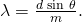Substituting known values yields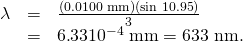Discussion

To three digits, this is the wavelength of light emitted by the common He-Ne laser. Not by coincidence, this red color is similar to that emitted by neon lights. More important, however, is the fact that interference patterns can be used to measure wavelength. Young did this for visible wavelengths. This analytical technique is still widely used to measure electromagnetic spectra. For a given order, the angle for constructive interference increases with, so that spectra (measurements of intensity versus wavelength) can be obtained.

Calculating Highest Order Possible

Interference patterns do not have an infinite number of lines, since there is a limit to how bigcan be. What is the highest-order constructive interference possible with the system described in the preceding example?

Strategy and Concept

The equation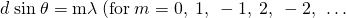describes constructive interference. For fixed values ofand, the largeris, the larger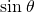is. However, the maximum value thatcan have is 1, for an angle of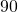. (Larger angles imply that light goes backward and does not reach the screen at all.) Let us find whichcorresponds to this maximum diffraction angle.

Solution

Solving the equation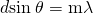forgives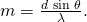Taking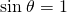and substituting the values ofandfrom the preceding example gives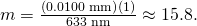Therefore, the largest integercan be is 15, or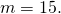Discussion

The number of fringes depends on the wavelength and slit separation. The number of fringes will be very large for large slit separations. However, if the slit separation becomes much greater than the wavelength, the intensity of the interference pattern changes so that the screen has two bright lines cast by the slits, as expected when light behaves like a ray. We also note that the fringes get fainter further away from the center. Consequently, not all 15 fringes may be observable.

# Section Summary

• Young’s double slit experiment gave definitive proof of the wave character of light.
• An interference pattern is obtained by the superposition of light from two slits.
• There is constructive interference when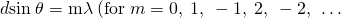, whereis the distance between the slits,is the angle relative to the incident direction, andis the order of the interference.
• There is destructive interference when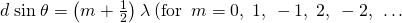.

# Conceptual Questions

Young’s double slit experiment breaks a single light beam into two sources. Would the same pattern be obtained for two independent sources of light, such as the headlights of a distant car? Explain.

Suppose you use the same double slit to perform Young’s double slit experiment in air and then repeat the experiment in water. Do the angles to the same parts of the interference pattern get larger or smaller? Does the color of the light change? Explain.

Is it possible to create a situation in which there is only destructive interference? Explain.

[link] shows the central part of the interference pattern for a pure wavelength of red light projected onto a double slit. The pattern is actually a combination of single slit and double slit interference. Note that the bright spots are evenly spaced. Is this a double slit or single slit characteristic? Note that some of the bright spots are dim on either side of the center. Is this a single slit or double slit characteristic? Which is smaller, the slit width or the separation between slits? Explain your responses.

This double slit interference pattern also shows signs of single slit interference. (credit: PASCO)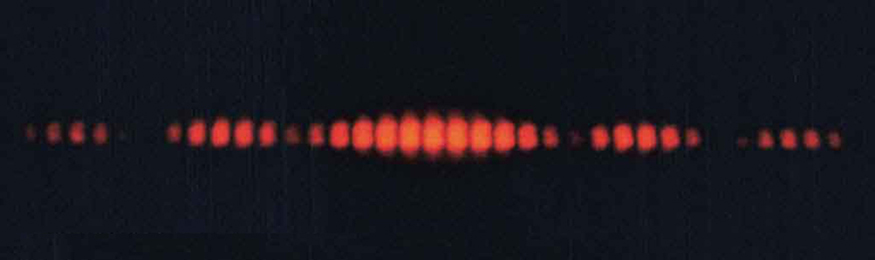# Problems & Exercises

At what angle is the first-order maximum for 450-nm wavelength blue light falling on double slits separated by 0.0500 mm?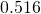Calculate the angle for the third-order maximum of 580-nm wavelength yellow light falling on double slits separated by 0.100 mm.

What is the separation between two slits for which 610-nm orange light has its first maximum at an angle of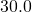?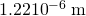Find the distance between two slits that produces the first minimum for 410-nm violet light at an angle of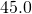.

Calculate the wavelength of light that has its third minimum at an angle ofwhen falling on double slits separated by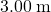. Explicitly, show how you follow the steps in Problem-Solving Strategies for Wave Optics.

600 nm

What is the wavelength of light falling on double slits separated by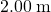if the third-order maximum is at an angle of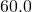?

At what angle is the fourth-order maximum for the situation in [link]?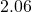What is the highest-order maximum for 400-nm light falling on double slits separated by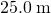?

Find the largest wavelength of light falling on double slits separated by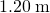for which there is a first-order maximum. Is this in the visible part of the spectrum?

1200 nm (not visible)

What is the smallest separation between two slits that will produce a second-order maximum for 720-nm red light?

(a) What is the smallest separation between two slits that will produce a second-order maximum for any visible light? (b) For all visible light?

(a) 760 nm

(b) 1520 nm

(a) If the first-order maximum for pure-wavelength light falling on a double slit is at an angle of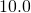, at what angle is the second-order maximum? (b) What is the angle of the first minimum? (c) What is the highest-order maximum possible here?

[link] shows a double slit located a distance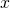from a screen, with the distance from the center of the screen given by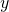. When the distancebetween the slits is relatively large, there will be numerous bright spots, called fringes. Show that, for small angles (where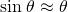, within radians), the distance between fringes is given by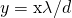.

The distance between adjacent fringes is, assuming the slit separationis large compared with.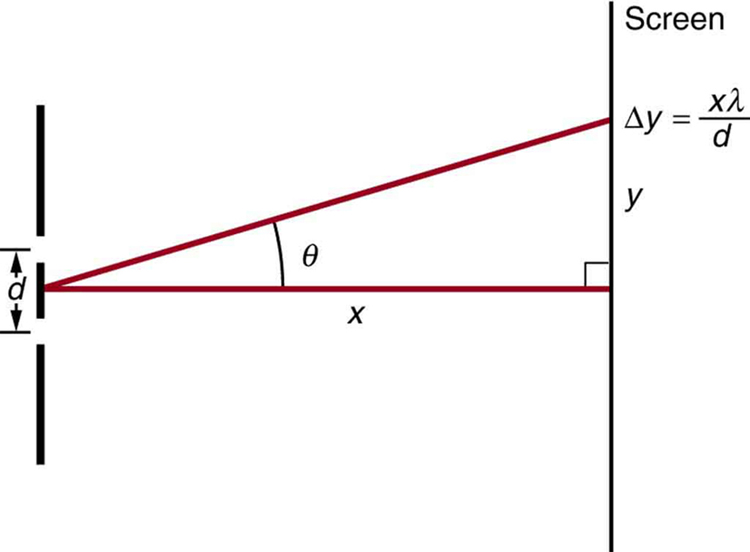For small angles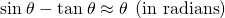.

For two adjacent fringes we have,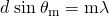and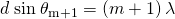Subtracting these equations gives

*** QuickLaTeX cannot compile formula:
\begin{array}{}d\left(\text{sin}\phantom{\rule{0.25em}{0ex}}{\theta }_{\text{m}+1}-\text{sin}\phantom{\rule{0.25em}{0ex}}{\theta }_{\text{m}}\right)=\left[\left(m+1\right)-m\right]\lambda \\ d\left({\theta }_{\text{m}+1}-{\theta }_{\text{m}}\right)=\lambda \\ \text{tan}\phantom{\rule{0.25em}{0ex}}{\theta }_{\text{m}}=\frac{{y}_{\text{m}}}{x}\approx {\theta }_{\text{m}}⇒d\left(\frac{{y}_{\text{m}+1}}{x}-\frac{{y}_{\text{m}}}{x}\right)=\lambda \\ d\frac{\Delta y}{x}=\lambda ⇒\Delta y=\frac{\mathrm{x\lambda }}{d}\end{array}

*** Error message:
Missing # inserted in alignment preamble.
leading text: $\begin{array}{} Missing$ inserted.
leading text: $\begin{array}{}d\left Missing$ inserted.
leading text: ...\left[\left(m+1\right)-m\right]\lambda \\ d
Missing $inserted. leading text: ...[\left(m+1\right)-m\right]\lambda \\ d\left Missing$ inserted.
leading text: ...\theta }_{\text{m}}\right)=\lambda \\ \text
Missing $inserted. leading text: ...ext{tan}\phantom{\rule{0.25em}{0ex}}{\theta Extra }, or forgotten$.
leading text: ...t{tan}\phantom{\rule{0.25em}{0ex}}{\theta }
Missing } inserted.
leading text: ...frac{{y}_{\text{m}}}{x}\right)=\lambda \\ d
Extra }, or forgotten \$.
leading text: ...frac{{y}_{\text{m}}}{x}\right)=\lambda \\ d
Missing } inserted.
leading text: ...frac{{y}_{\text{m}}}{x}\right)=\lambda \\ d



Using the result of the problem above, calculate the distance between fringes for 633-nm light falling on double slits separated by 0.0800 mm, located 3.00 m from a screen as in [link].

Using the result of the problem two problems prior, find the wavelength of light that produces fringes 7.50 mm apart on a screen 2.00 m from double slits separated by 0.120 mm (see [link]).

450 nm

## Glossary

coherent
waves are in phase or have a definite phase relationship
constructive interference for a double slit
the path length difference must be an integral multiple of the wavelength
destructive interference for a double slit
the path length difference must be a half-integral multiple of the wavelength
incoherent
waves have random phase relationships
order
the integerused in the equations for constructive and destructive interference for a double slit